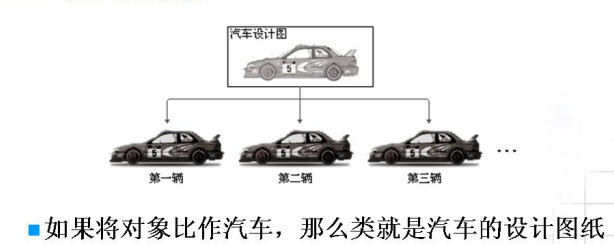1、類和物件的概念

1>把一類事物的靜態屬性和動態可以執行的操作組合在一起所得到的這個概念就是類
2>類的一個個體就是物件,物件是具體的,實實在在的事物
3>物件是特徵與技能的結合體，其中特徵和技能分別對應物件的資料屬性和方法屬性。2、訪問控制符的具體用法

1>在一個類的內部,所有的成員之間彼此之間都可以進行相互訪問,訪問控制符是透明的,失效的,共有的可以呼叫私有的,私有的可以呼叫共有的
2>在一個類的外部,只有其私有成員不可以通過類物件的方式進行訪問,共有成員和保護型的成員都可以通過這種方式進行訪問,換言之,在一個類的外部,通過類物件名.私有成員的方式是無法訪問該物件中的私有成員的

package App;

public class App1
{
public static void main(String[] args2)
{
A aa = new A(2,3);
aa.fun();
}
}

class A
{
private int i;
private int j;
public A(){}
public A(int i,int j){this.i = i;this.j = j;}
public void fun()
{
System.out.println(this.i+"\t"+this.j);
}
}

2   3
 3、建構函式的概念以及相應的作用

1>建構函式的名字和類的名字相同
2>建構函式沒有返回值
3>建構函式可以有形式引數,也可以沒有形式引數
4>一個類中可以有多個建構函式

package App;

public class App1
{
public static void main(String[] args2)
{
A aa =  new A();
A bb = new A(2,3);
}
}

class A
{
private int i;
private int j;
public A(){System.out.println("自動呼叫方法!");}
public A(int i,int j)
{
this.i = i;
this.j = j;
System.out.println("自動呼叫方法!");
}
public void fun()
{
System.out.println(this.i+"\t"+this.j);
}
}

 4、區域性變數與類屬性初始化的問題

1>在Java中,如果是個區域性變數(在一個函式內部定義的變數或者函式的形式引數),必須進行初始化,否則會出錯
2>類中的屬性,如果沒有進行初始化,將會被自動賦值.

package App;

public class App1
{
public static void main(String[] args2)
{
System.out.println(new A().i);//輸出為0
System.out.println(new A().j);//輸出為0
int i;
System.out.println(i);
}
}

class A
{
public int i;
public int j;
public A(){}
public A(int i,int j)
{
this.i = i;
this.j = j;
}
}

Exception in thread "main" java.lang.Error: Unresolved compilation problem:
The local variable i may not have been initialized
 5、函式的過載（C語言也支援）

1>形式引數個數的不同
2>形式引數資料型別的不同
3>形式引數順序的不同

package App;

public class App1
{
public static void main(String[] args2)
{
System.out.println(String.valueOf(66.6));
System.out.println(String.valueOf(66.6f));
System.out.println(String.valueOf(66));
System.out.println(String.valueOf(66L));
}
}

66.6
66.6
66
66

public class App1
{
public static void main(String[] args)
{
Scanner scanner = new Scanner(System.in);
int a = scanner.nextInt();
int b = scanner.nextInt();
int c = scanner.nextInt();

System.out.println(max(10,20));
System.out.println(max(10,20,30));
}
public static int max(int i,int j)
{
return i+j;
}
public static int max(int i,int j,int k)
{
return i+j+k;
}
}
 6、this關鍵字的用法

package App;

public class App1
{
public static void main(String[] args2)
{
A  aa = new A(2,3);
System.out.println(aa.toString());
}
}

class A
{
public int i;
public int j;
public A(){}
public A(int i,int j)
{
this.i = i;
this.j = j;
}
public String toString()
{
return this.i + "\t" + this.j;
}
}

2   3
•  7、利用static統計一個類到底產生出多少個類物件(實用程式)

1>如果一個成員前面加一個static,意味著這個成員不再是屬於某個類物件的,而是被n個物件所共有的,即靜態成員屬於類本身的,由作業系統只分配一塊記憶體空間,大家共同使用這一塊記憶體空間,對同一個變數進行操作

2>靜態的屬性和方法屬於類本身的,可以通過類名的方式進行訪問
3>建立完類物件之後,系統首先為這個物件分配好記憶體空間,然後由類自動呼叫相應的建構函式

package App;

public class App1
{
public static void main(String[] args2)
{
A aa = new A();
A bb = new A(2,3);
A cc = new A();
A dd = new A();
A ee = new A(2,3);
A ff = new A();
System.out.println(A.getCount());
}
}

class A
{
private int i;
private int j;
private static int num = 0;//分配一塊記憶體空間
public A()
{
num ++;
}
public A(int i,int j)
{
this.i = i;
this.j = j;
num++;
}
public static int getCount()
{
return num;//類內部所有成員都可以進行相互訪問
}
}

6
•  8、static單態涉及模式::要求一個類只能生成一個類物件(實用)

package App;

public class App1
{
public static void main(String[] args2)
{
A aa = A.getObject();
A bb = A.getObject();
A cc = A.getObject();//返回的是同一個類物件
if(aa == bb )
System.out.println("true");
if(aa == cc )
System.out.println("true");
if(bb == cc )
System.out.println("true");
}
}

class A
{
private static A  aa = new A();
private A(){}
public static A getObject()
{
return aa;
}
}

true
true
true
 9、多型的概念

package App;

public class App1
{
public static A  aa ;
public static void main(String[] args2)
{
aa = new A(2,3); //指向父類物件
aa.fun();
aa = new B(2,3,4); //指向子類物件
aa.fun();
}
}

class A
{
public int i;
public int j;
public A(){}
public A(int i,int j)
{
this.i=i;
this.j=j;
}
public void fun()
{
System.out.println(this.i+"\t"+this.j);
}
}
class B  extends A
{
public int k;
public B(){}
public B(int i,int j,int k)
{
this.i = i;
this.j = j;
this.k = k;
}
public void fun()
{
System.out.println(this.i+"\t"+this.j+"\t"+this.k);
}
}

2   3
2   3   4
•  10、A類繼承了B類意味著什麼?

1>若A類繼承了B類,則各種修飾符相應的也被繼承了過來
2>若A類繼承了B類,則繼承過來的成員相應的也就屬於A類本身了

 11、同包繼承許可權的問題

package App;

public class App1
{
public static void main(String[] args2)
{
A aa = new A();
System.out.println(aa.i);
System.out.println(aa.j);
//System.out.println(aa.k);父類的私有成員無法被子類繼承
aa.fun();
}
}

class A
{
public int i;
protected int j;
private int k;
public A(){}
public A(int i,int j,int k)
{
this.i = i;
this.j = j;
this.k = k;
}
public void fun()
{
System.out.println("AAAA");
}
}
class B  extends A
{
public void fun()
{
System.out.println("AAAA");
}
}

0
0
AAAA
 12、super關鍵字的使用

super關鍵字產生的原因:子類可以繼承父類除私有成員以外的所有成員,但是子類永遠無法繼承父類的構造方法,在子類的構造方法中通過使用super關鍵字來呼叫父類的構造方法
super語句的三個注意事項:
1>每個子類構造方法中的第一條語句,都是隱含的呼叫super()語句
2>如果顯示的寫出super語句,則必須保證該語句是子類構造方法中的第一條語句,並保證父類中有相應引數的構造方法,否則會出錯
3>通過super呼叫父類的構造方法只放在子類的構造方法中,不能放在普通方法中,並且該語句只能放在子類構造方法中的第一條語句

package App;

public class App1
{
public static void main(String[] args2)
{
B  bb = new B(1,2,3,4);
System.out.println(bb.i+"\t"+bb.j+"\t"+bb.k+"\t"+bb.l);
}
}

class A
{
public int i;
public int j;
public int k;
public A(){}
public A(int i,int j,int k)
{
this.i = i;
this.j = j;
this.k = k;
}
}
class B  extends A
{
public int l;
public B(){}//隱含著呼叫A()
public B(int i,int j,int k,int l)
{
//     this.i = i;
//     this.j = j;
//     this.k = k;
super(i,j,k);//理解為呼叫A(i,j,k);
this.l = l;
}
}

1   2   3   4
 13、方法重寫的概念

[若兩個函式只有函式返回值不同,則編譯時會出錯]

package App;

public class App1
{
public static void main(String[] args2)
{
A aa =  new A(1,2,3);
System.out.println(aa.toString());
}
}

class A
{
public int i;
public int j;
public int k;
public A(){}
public A(int i,int j,int k)
{
this.i = i;
this.j = j;
this.k = k;
}
public String toString()
{
return this.i+"\t"+this.j+"\t"+this.k;//重寫Object類中的toString()方法
}
}

1   2   3
 14、多型的兩個注意事項

1>通過父類的引用只能訪問子類物件從父類繼承過來的成員,但是不能訪問子類物件所特有的成員(父類引用強制轉化為子類的引用的原因)
2>父類的引用永遠不可能直接賦給子類引用,否則會出錯,只有在父類的引用本身指向的就是子類物件的時候,才可以將父類引用強制轉化為子類的引用(其它情況下不允許將父類的引用強制轉化為子類的引用,否則執行的時候會出錯)

package App;

import java.awt.List;
import java.util.ArrayList;
import java.util.Collections;

public class App1
{
public static ArrayList aa;
public static void main(String[] args2)
{
aa = new ArrayList<Student>();
Collections.sort(aa);
System.out.println(aa);
}
}

class  Student  implements Comparable
{
public String name;
public double score;
public Student(){}
public Student(String name,double score)
{
this.name = name;
this.score = score;
}
public int compareTo(Object obj)//父類的引用obj此時指向了子類的物件Student
{
Student cc = (Student)obj;//在父類的引用指向子類物件的前提下,將父類的引用強制轉化為子類的引用
/**之所以要將父類的引用強制轉化為子類的引用,主要是因為父類的引用不能呼叫子類所特有的成員****/
if(this.score>cc.score)
return 1;
else if(this.score < cc.score)
return -1;
else
return 0;
}
public String toString()
{
return this.name + "\t" + this.score;
}
}

[wangwu 66.6, lisi  88.8, zhangsan  98.8]
 15、抽象類與抽象方法

1>沒有執行體的方法叫做抽象方法,抽象方法要求末尾必須得加分號,前面必須得加abstract進行修飾

2>抽象方法通過子類的實現可以變成普通的方法
3>抽象方法不存在所謂重寫的問題,卻存在著實現的問題
4>含有抽象方法的類一定是抽象類,但是抽象類不一定含有抽象方法,此時也就沒有什麼意義了

abstract class A//抽象類
{
public int i;
public int j;
public int k;
public A(){}
public A(int i,int j,int k)
{
this.i = i;
this.j = j;
this.k = k;
}
public abstract void dat();//抽象方法
public void fun()//普通方法
{
System.out.println(i+j+k);//方法體
}
}
 16、介面的概念

interface A
{
int i = 5;//數值型常量<--> public static final int i = 5;
void fun();//抽象方法<-->  public abstract void fun();
}
 17、介面與抽象類的區別

1>介面可以近似的當做一個抽象類,但是卻不是抽象類,更不是類,介面只能被一個類實現,但是抽象類卻可以被一個類繼承
2>介面中的方法不允許有方法體,但是抽象類中的方法卻允許有方法體
3>介面可以實現多繼承,但是抽象類只能實現單繼承

 18、final關鍵字